# Elevation Element

## Elevation Element General Description & Quick Guide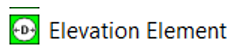Elevation Element (gravitational orifice) is similar to Orifice element is used to calculate orifice flow including the effect of gravity and rotation. Elevation Element uses an input of Orifice mechanical Area/Diameter & Loss parameters and Gravitational effects (Inlet & Exit Heights). Loss Parameter can be Cd (Coefficient of discharge) or Incompressible Loss Coefficient (K). Elevation element can be found under both “Compressible Gas Elements” & “Incompressible Liquid Elements” section.

## Elevation Element Inputs

Table of the inputs for the Elevation Element.

## Elevation Element Theory Manual

The flow function written to define the flow through an elevation element in terms of upstream total pressure, upstream total temperature, downstream static pressure and cross-sectional flow area. Since the flow function is derived with the assumption of isentropic flow, some sort of empirical correction is required to account for the irreversible losses encountered in the Orifices. This is usually contained in form of a discharge coefficient or Loss coefficient, included in the working definition of flow function and represents the ratio of actual (empirical) to ideal (isentropic) flow rates.

 Nomenclature: W: Mass flow rate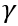: Specific heat Ratio A: Orifice mechanical area R: Gas Constant CD: Coefficient of Discharge Ts: Static Temperature K: Incompressible Loss Coefficient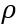: Density Tt: Total Temperature MN: Vena Contracta Mach Number Pt: Total pressure Cp: Specific Heat Ps: Static pressure gc: Gravitational Constant JLC = 778.169

Discharge Loss Coefficient Calculations

Loss Mode: Cd – Compressible Loss Coefficient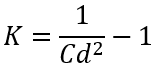Loss Mode: K – Incompressible Loss Coefficient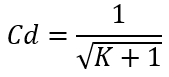Flow Rate Calculations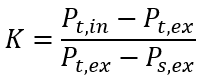These equations can be used to derive an expression for mass flow rate, .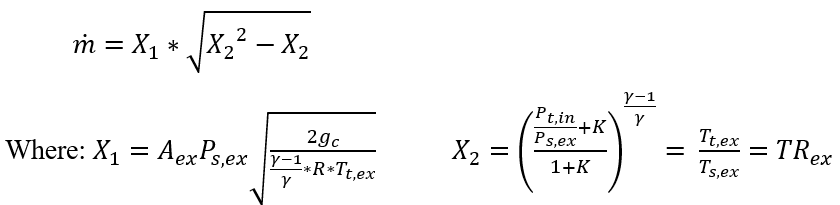In the above equations, the corrected pressures (for the effects of Gravity & Pumping) will be used, which are defined below

Compressible and Incompressible Gas Conditions:

Inlet Conditions: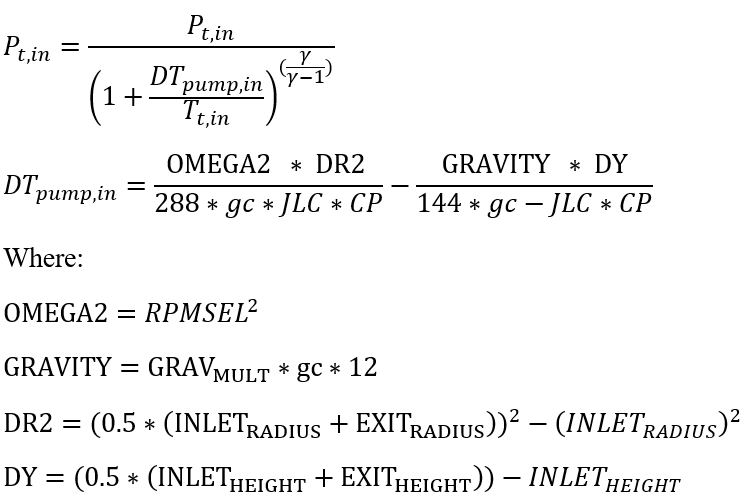Exit Conditions: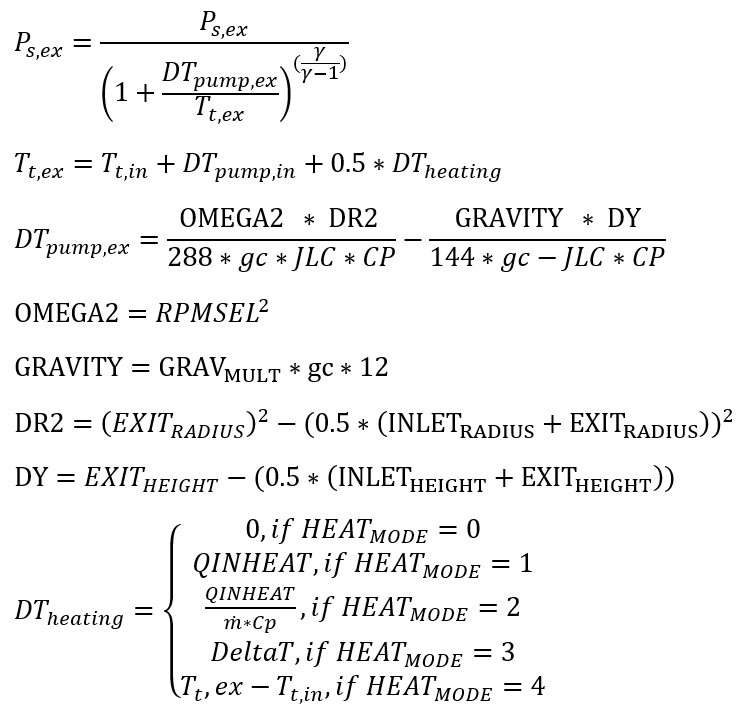Incompressible Liquid Conditions:

Inlet Conditions: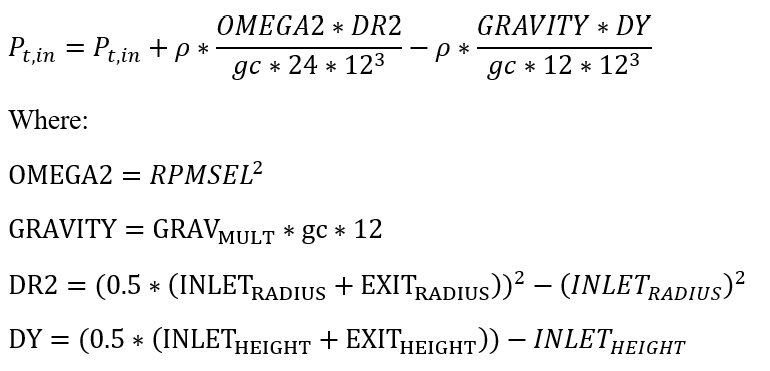Exit Conditions: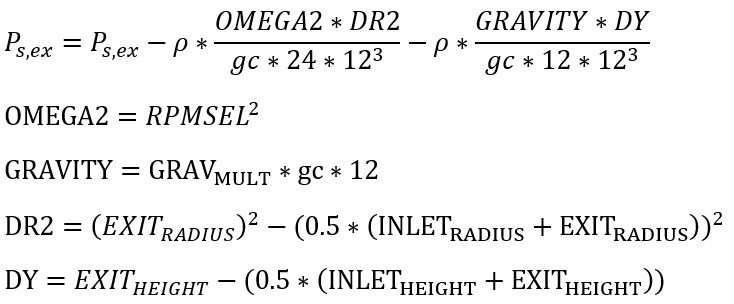## Elevation Element Outputs

The following listing provides details about conventional orifice output variables.

Name Description Units

FLOWS:

FLOW: %WREF & MASSFLOWRATE

Flow rates in %WREF and [PPS]or [kg/s] (None) & (PPS or kg/s)
SOLVER_STAT Information on status of the elevation element solution Flag
FLUID: Fluid equation type information Flag
CHOKED_FLOW_SOLVER_STAT Convergency information Flag
NEWTON_ITERS: How many iterations before non-convergence Flag
ERROR: Error value if the solver does not converge to solve elevation element Unitless
DQ_IN

Portion of Ustrm Chamb. Dyn. Head Lost

(Usually an echo of the user input unless modified inside Flow Simulator.)

Flag

Axial (K_EXIT_Z)

Tangential (K_EXIT_U)

Exit K Loss

(Usually an echo of the user input unless modified inside Flow Simulator.)

Unitless
ELEMENT_THETA

Tangential Angle

(Usually an echo of the user input but converted to radians.)

ELEMENT_PHI

(Usually an echo of the user input but converted to radians.)

REL_INLET_ANGLE It is a relative inlet angle calculated based on upstream chamber velocity Deg
ELEMENT_RPM

RPM (Rotor index)

(Usually an echo of the user input unless modified inside Flow Simulator.)

RIN

(Usually an echo of the user input unless modified inside Flow Simulator.)

in, m
REX

(Usually an echo of the user input unless modified inside Flow Simulator.)

in, m
GRAV_MULT Gravity Multiplier
INLET_HEIGHT

Inlet Height

(Usually an echo of the user input unless modified inside Flow Simulator.)

in,m
EXIT_HEIGHT

Exit Height

(Usually an echo of the user input unless modified inside Flow Simulator.)

in,m
ELEMENT_AREA

Cross-sectional area.

(Usually an echo of the user input unless modified inside Flow Simulator.)

inch2, m2
CD

Discharge coefficient.

(Usually an echo of the user input unless modified inside Flow Simulator.)

(fraction)
K

Calculated from the discharge coefficient using equation

(unitless)
EXIT_AREA

Exit area used for calculating exit conditions of the orifice element. This output is only printed when an exit area is used (EXIT_AREA>0). A default value of 0 has no effect on exit conditions.

(Output is an echo of the user input.)

inch2, m2
PTS Driving pressure relative to the rotational reference frame (i.e. rotor) at the restriction inlet. psi, mPa
PTEX Total pressure relative to the rotational reference frame (i.e. rotor) at the restriction exit including supersonic effects. psi, mPa
PSEX

Static pressure relative to the rotational reference frame (i.e. rotor) at the restriction exit.

Limited by critical pressure ratio for supersonic flows.

psi, mPa
PSEB Effective sink (static) pressure downstream of the restriction. psi, mPa
TTS Total temperature of fluid relative to the rotational reference frame (i.e. rotor) at the restriction inlet. deg F, K
VCMN Fluid Mach number relative to the rotational reference frame (i.e. rotor) at the vena contracta. (unitless)
VXA Fluid velocity relative to the rotational reference frame (i.e. rotor) at the restriction exit before heat input (QIN) effects. ft/s, m/s
EXMN Fluid Mach number relative to the rotational reference frame (i.e. rotor) at the restriction exit before heat input (QIN) effects. (unitless)
QIN

Heat input.

Positive values indicate heat added to the fluid; negative values indicate heat removed.

BTU/s, W
DT Change in total temperature relative to the rotational reference frame (i.e. rotor) due to heat input (QIN). deg F, K
TEX Total temperature relative to the rotational reference frame (i.e. rotor) at the restriction exit. deg F, K
VEX Fluid velocity relative to the rotational reference frame (i.e. rotor) at the restriction exit including heat input (QIN) effects. ft/s, m/s
VABS Magnitude of the fluid total absolute velocity ft/s, m/s
VTAN_ABS Magnitude of the fluid absolute tangential velocity ft/s, m/s
VAXIAL Magnitude of the fluid axial velocity ft/s, m/s